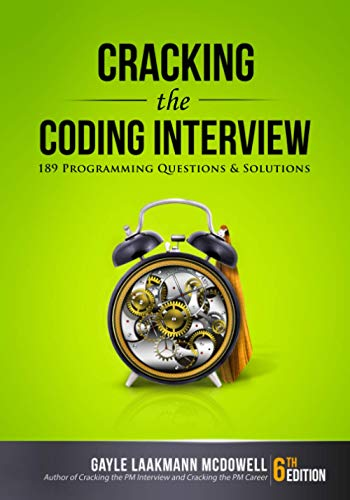# The Ant Problem

Question: Three ants are sitting at the three corners of an equilateral triangle. Each ant starts randomly picks a direction and starts to move along the edge of the triangle. What is the probability that none of the ants collide?

Answer: So let’s think this through. The ants can only avoid a collision if they all decide to move in the same direction (either clockwise or anti-clockwise). If the ants do not pick the same direction, there will definitely be a collision. Each ant has the option to either move clockwise or anti-clockwise. There is a one in two chance that an ant decides to pick a particular direction. Using simple probability calculations, we can determine the probability of no collision.

P(No collision) = P(All ants go in a clockwise direction) + P( All ants go in an anti-clockwise direction) = 0.5 * 0.5 * 0.5 + 0.5 * 0.5 * 0.5 = 0.25

Liked this post? Please Digg or Stumble it.

If you're looking for some serious preparation for your interviews, I'd recommend this book written by a lead Google interviewer. It has 189 programming questions and solutions:## 13 Responses

1.David o says:

Ants also leave a scent behind that other ants follow whatever direction. The first ant moves the other 2 will follow

2.David o says:

They won’t. Collide if their from the same colony ants won’t Collide unless their at war trick question!

3.fun says:

nobody here took into account speed. what if all ants are moving in same direction, but one or more have different speeds.

4.nishant says:

but it collision wont depends on speed of ants??????/

5.cancan says:

This blog was… how do I say it? Relevant!

! Finally I’ve found something that helped me. Thanks a lot!

6.Yusuf says:

7.Adam G. Freeman says:

Awesome!!!!

8.sigma says:

2^3 cases = 8 cases
2 correct cases

P(correct cases) = 2 / 8 = .25

9.Tal Achituv says:

An alternative way to look at it is:

All ants have to choose the same direction.
It doesn’t matter which direction ant #1 picked, the only thing that matters is that ants #2 & #3 pick the same as #1.

So the solution is P=[1/(n-1)^2] = [1/4]

In other words, the probability of ant #1 picking the “correct direction” is 1, Since there is no “wrong direction” at that point.
Each subsequent ant has a P=[1/2] of picking the “correct direction” (at this point there is a “correct” direction, which is whichever direction #1 picked.)

P(no collision) = 1 * 0.5 * 0.5 = 1/4

10.Akshitha says:

super correct!

11.sanjeev says:

12.Pavan says:

lets do it in the other way:) the probability of they not getting collided is one less than all the probability of they getting collided,which is 3/4.
Every ant will have 0.5 probability of choosing a particular path and so the probability of one ant getting collided is 0.5*0.5. the total probability of 3 ants getting collided in different cases is 3*0.5*0.5,which is 3/4. hence the result 1/4

13.Dan says:

another way to find the answer:

lets assume all three ants are looking towards the center. They will not collide if all of them are moving towards left or towards right. Even if one of the ants starts to move to the other direction there will be a collision. There are the following eight optio
RRR
LLL
RLL
RLR
RRL
LRL
LRR
LLR

The ants will not collide in case of LLL and RRR so the probability of not colliding is:
2/8 = 0.25

XHTML: These are some of the tags you can use: `<a href=""> <b> <blockquote> <code> <em> <i> <strike> <strong>`